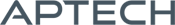SSATS 2.0: State Space Aoki Time Series

SSATS 2.0: State Space Aoki Time Series

Availability: Out of stock

SSATS 2.0 is a set of preprogrammed GAUSS procedures that perform all the tasks necessary to and associated with the specification, estimation, and forecasting of multivariate state space time series models. SSATS will be useful to any researcher who...
Overview

J. Dorfman

The following product is developed by J. Dorfman, a third party developer, for use with GAUSS. Technical support is provided directly through the developer.

State Space Aoki Time Series

SSATS 2.0 is a set of preprogrammed GAUSS procedures that perform all the tasks necessary to and associated with the specification, estimation, and forecasting of multivariate state space time series models. A standard state space model takes the form:

yt = Cz t + et (observation equation)
zt+1 = Az t + Bet (state equation)

where yt is an (m x 1) vector of the time series to be modeled and/or forecast, z tis the (n x 1) state vector, e t is an (m x 1) vector of stochastic innovations (error terms), and A, B, and C are parameter matrices to be estimated.

Masanao Aoki developed a particularly successful algorithm to estimate such models based on the balanced representation and relying heavily on results from linear systems theory. SSATS 2.0 will let a researcher easily begin to implement the techniques laid out in Aoki's book, State Space Modeling of Time Series (Springer-Verlag, 1987, 1990).

SSATS will be useful to any researcher who is interested in empirical work on multivariate dynamic systems. SSATS is a valuable tool for anyone involved in the specification, estimation, and forecasting of multivariate (or univariate) time series models. The procedures can be used on their own, combined into a single command program, or used selectively in conjunction with other time series methods to aid in specification or forecast evaluation.

Platform: Windows, Mac, Linux

Requires: GAUSS Mathematical & Statistical System/GAUSS Engine v3.2 and above.

Features

SSATS 2.0 provides procedures to easily accomplish such tasks as:

• Scale and center data prior to estimation
• Choose the model specification (model order of the time series),
• Estimate the model coefficients A, B, and C
• Estimate covariance matrices of parameter matrices, data series, errors, and states
• Evaluate model specification with diagnostic tools
• Produce in-sample and out-of-sample forecasts
• Evaluate forecasting performance including a variety of summary statistics.

All of the forecasting evaluation procedures can be used with forecasts generated by any methods; they are not restricted to use with state space models. Similarly, the model specification procedures and statistical tests included can be used to identify the model order of a time series even if the researcher then estimates a VAR or VARMA model instead of a state space model.

The SSATS 2.0 procedure module comes with:

• 19 procedures
• A complete user's guide containing descriptions and examples for all procedures
• A primer on state space models, the Aoki estimation algorithm, and tips and guidance on how to successfully model and forecast multivariate time series using state space models
• A sample program showing how to combine the procedures into a complete implementation of the procedures to specify a model, estimate it, produce forecasts, and evaluate the model's performance
• A sample data set and demo output to allow researchers to insure that the programs are working properly on their systems.

Product Inquiry

5 + 5 = enter the result ex:(3 + 2 = 5)

﻿